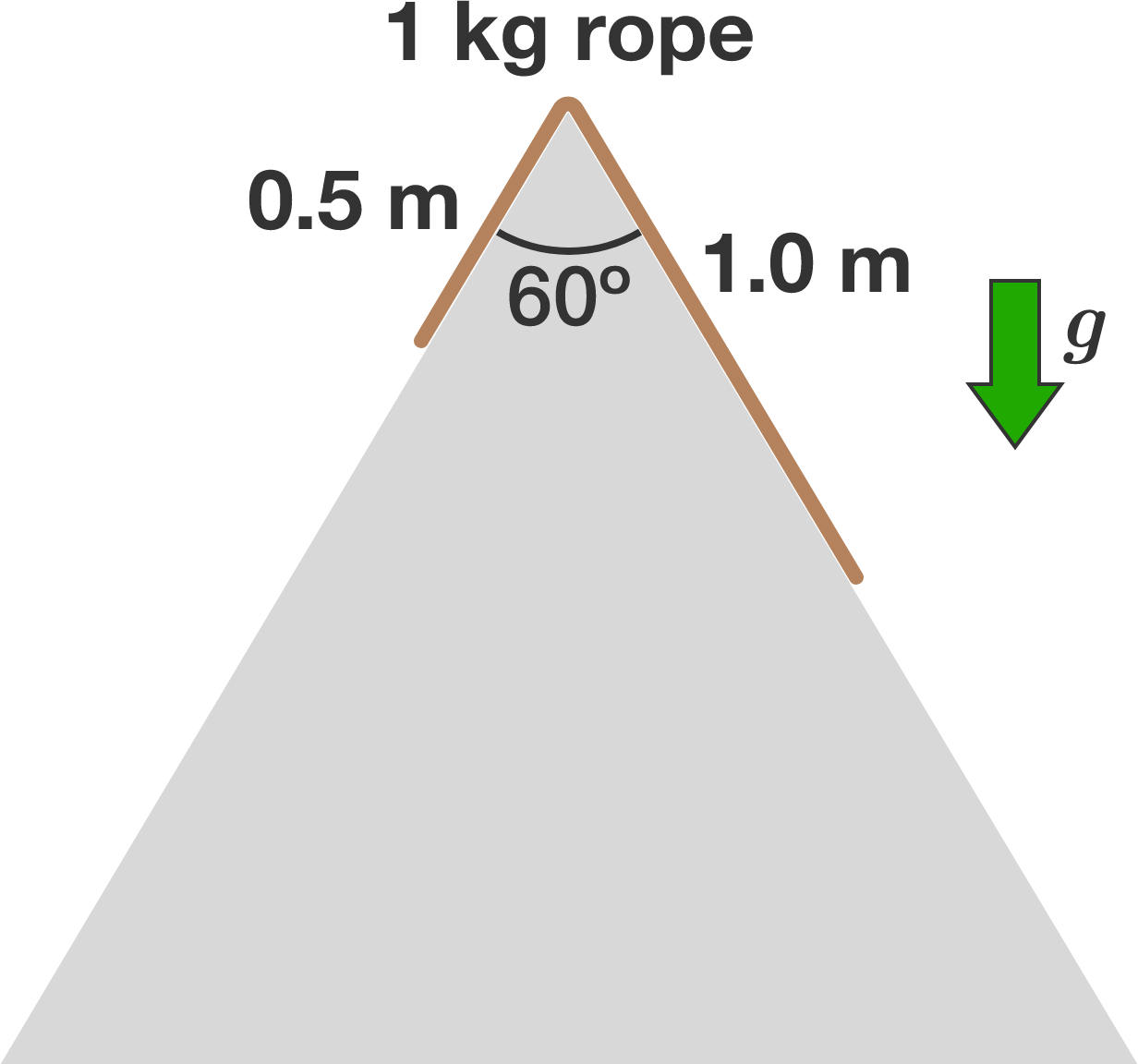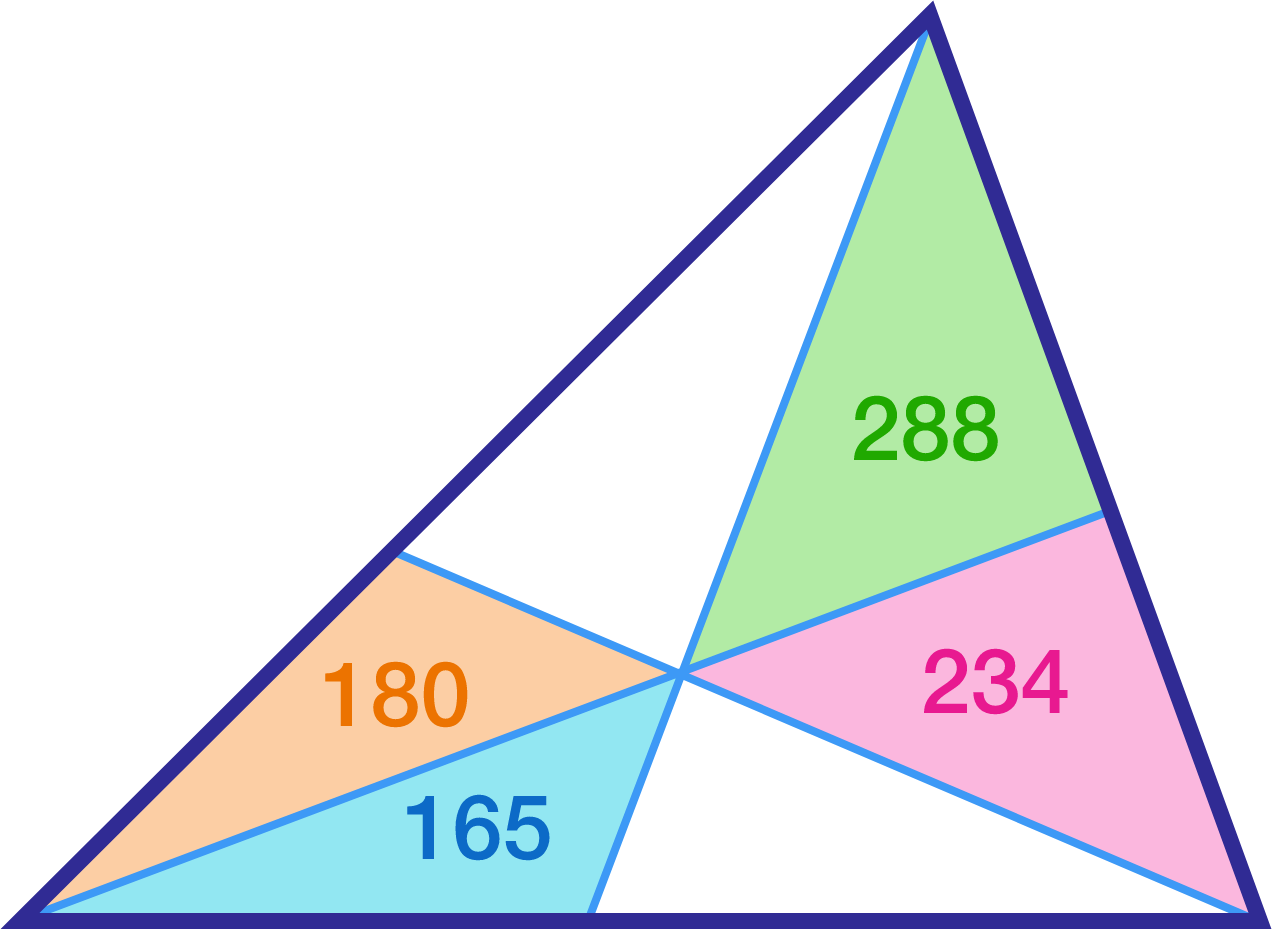# Problems of the Week

Contribute a problem

$\large f(x) =\sqrt [ 3 ]{ 1-x } +\sqrt [ 3 ]{ x+3 }, \,\,x\in \mathbb{R}$

Suppose $L$ is the maximum value of the function $f$. Find the value of $\lfloor 10000L\rfloor$.


Notation: $\lfloor \cdot \rfloor$ denotes the floor function.

$x^2\big(y^3+z^3\big)=315(xyz+7)$

Let $(x_1, y_1, z_1), (x_2, y_2, z_2), \ldots , (x_n, y_n, z_n)$ be all of the solutions to the equation above, where $x, y, z$ are positive integers. Find $\displaystyle \sum_{k=1}^{n} (x_k+y_k+z_k)$.A $\SI{1}{\kilo\gram}$ rope of length $\SI{1.5}{\meter}$ slides down a frictionless double ramp in the shape of an equilateral triangle, as shown in the diagram above. At time $t = 0$, the rope is at rest with $\SI{1}{\meter}$ of its length on the right side of the ramp.

How much time (in seconds, to 3 decimal places) elapses before all of the rope is on the right side?


Details and Assumptions:

• The downward gravitational acceleration is $\SI[per-mode=symbol]{10}{\meter\per\second\squared}.$

Let $x, y,$ and $z$ be lengths of the sides of a triangle such that $x + y + z = 2$.

The range of $xy + yz + zx - xyz$ is $(m, n ]$.

If $m+n = \frac{a}{b}$, where $a$ and $b$ are coprime positive integers, what is $a+b$?

A math teacher had drawn on the blackboard a very carefully scaled figure of a triangle divided into six smaller triangles with three straight cuts as follows:and he proceeded to write in the integer area of each of the six smaller triangles. But as he wrote down the area of the fourth triangle (as shown in this graphic), a bright student raised his hand and said,

"Mr. teacher, but that's wrong, you made a mistake with that number you just wrote!"

The teacher looked at his notes and realized his error, so he rubbed out the last digit of the area of the fourth triangle, put in the correct digit, and then continued to write in the areas for the fifth and sixth triangles.

What was the total area of the big triangle?

×